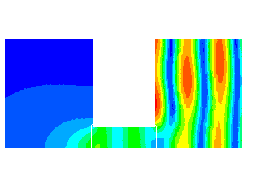## PAFEC VibroAcoustics - Architectural

 Acoustic finite and boundary elements solve the Helmholtz equations exactly, within the discretization error. Thus effects such as defraction are naturally modelled. It is also possible to model interactions with structural objects such as windows. However, the element size required is proportional to the wavelength, therefore as the number of elements increases the problem becomes computationally larger. Thus FE and BE techniques can be used to analyse 'small rooms' Areas of localised design, such as diffusers or apertures can benefit from finite and boundary element analyses. Axisymmetic analysis of a cylindrical aperature in a rigid baffle connecting two half spaces. Each half space is bounded by an infinite rigid baffle, with the aperature at the centre. A plane wave is incident on the baffle in the right hand space. A combination of acoustic finite and boundary elements are used to model this problem.Pressure amplitide distribution at 2250Hz.Animated pressure amplitide distribution at 2250Hz.
 Back Example: